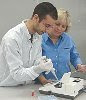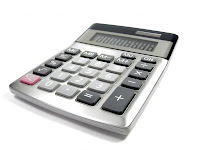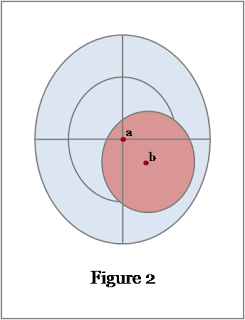## Friday, 8 May 2009

### "I'm a trainer? But that's not in my job description. Is it?"

LEARNING AT WORKTraining know-how applied to laboratory scienceAre you a trainer?
If you work in a science laboratory environment then you probably are. In most working environments it is normal practice for an experienced member of staff to show new and less experienced staff how to perform common work related tasks. This practice is very suited to a laboratory setting due to the large range of tasks that need to be learned by a new starter. These include learning about: the range of procedures to be followed; the ways of working; the operation and calibration of equipment and instrumentation; computer software applications used in the laboratory, etc. Sometimes this type of training is provided within a formal framework, where individuals are assigned to maintain and train others on a specific piece of equipment or analytical technique, but sometimes it is simply a case of “will you show John how to use the Karl Fischer?” An initial training session may be followed up with support for the learner as they gain competence in the task in question.

On-the-job training has clear advantages for the laboratory if it is well implemented; it is cost effective, flexible, and the learner is doing the job while they learn. It is also good for the learner; they are trained by someone with a good working knowledge of the task and implementation of the learning is usually possible immediately. But what about the trainer? Is training others a welcome and enjoyable responsibility, or an unenviable extra duty to try to fit into a busy work schedule? This depends on how the individual feels about being a trainer. Some people enjoy it more than others and some find the whole concept very daunting .

Of course the benefits listed above only apply when the training being delivered is successful and effective. One of the problems when using in-house staff as trainers is that the quality of the training can be variable and the individuals involved are not always given guidance on how to plan and deliver training. The purpose of this series of articles is to provide advice and tips on the best way to approach a training event. The word ‘event’ is used here to signify any training programme, large or small, which needs to be implemented in your laboratory.

The implementation of training is usually described using the training cycle shown below. There are four main stages:

1. Identify the learning needs
2. Design the training
3. Deliver the training
4. Evaluate the training

Next time we will look at each of these stages in more detail to give you an overview of what you need to do to implement your training event.## Wednesday, 6 May 2009

### Free HPLC calculator from Thermo

FREE STUFF ONLINEYou can find numerous useful resources for pharmaceutical analysis on the internet which don’t cost anything. Suppliers and manufacturers of analytical equipment and instrumentation need to position themselves as experts so that they can gain your trust in their products. They do this by providing a range of useful resources relating to their product area; often these resources are available online.

An example of a useful resource for HPLC is the method development calculator from Thermo, available at:
http://www.hplctransfer.com/

This calculator allows you to transfer a HPLC method to a different set of column dimensions. You enter the current method conditions in the left hand column and the new column dimensions in the right hand column. Then the calculator will work out the required flow rate, injection volume, predicted system pressure, gradient conditions and suggested equilibration time. You can also specify a flow rate and get adjusted values for the parameters listed above. There are two calculators, one for gradient and one for isocratic methods. The application is aimed at transferring standard HPLC methods to ultra high pressure conditions but the calculations hold for whatever transfer you require.

I like this calculator because it is well laid out and easy to use. I also like the fact that an explanation of all the equations used is provided. I think that this could be a useful tool to save time in your HPLC method development.

## Friday, 1 May 2009

### Error and uncertainty in analytical measurement - Part I

ANALYTICAL TOPICS

When analysing pharmaceuticals the objective of our efforts is to produce a result, an analytical measurement, which gives important information about the sample of interest. Examples are: the determination of impurities in an active pharmaceutical ingredient; and the assay of the amount of the active in a dosage form. In these examples the information obtained may be used to ensure that the product is safe and of high quality. It is vital that the analytical results obtained are reliable since they are used to make decisions which can have an impact on human health.

A reliable result does not mean that the analytical measurement is exactly equal to the actual value of the parameter being measured (the true value). We accept that the result is an estimation of the true value and that the difference is due to error and uncertainty introduced during the measurement process. However, for the result to be deemed reliable, we need to determine the magnitude of this difference between the analytical measurement and the true value. This requires a quantification of the error and/or uncertainty associated with the analytical method.

So what are error and uncertainty, and what is the difference between them?Error is defined by ISO  as “the result of a measurement minus a true value of the measurand”, (where the measurand is the “particular quantity subject to measurement”). In Figure 1 the true value of an analytical measurand is shown by a, the centre of the target. An analytical measurement is shown by b. The difference between the true value and the measured value is given by x. Therefore the error for the measurement is equal to x. Error is a single value for each analytical measurement. In theory a known value for error can be used to correct the result.

Error in analytical measurement is regarded as being made up of two different types: random error and systematic error. Random error is caused by the many uncontrollable variables that are an inevitable part of every chemical or physical measurement. This type of error can usually be reduced by multiple measurements. Systematic errors have a definite cause and are of the same value for a number of replicates analysed in the same way. They are independent of the number of measurements and therefore cannot be reduced by increasing the number of measurements. A further type of error is a spurious error, or blunder, which invalidates a measurement. This can occur when the person performing the analysis makes a mistake or an instrument fails.Uncertainty is defined by ISO  as a “parameter associated with the result of a measurement, that characterises the dispersion of the values that could reasonably be attributed to the measurand.” This parameter may take the form of some multiple of the standard deviation, or confidence limits relating to an analytical measurement. It is usually expressed as a range within which the true value is known to fall. The uncertainty may be determined for a particular analytical method or procedure and thus applied to all results obtained for the method, provided that it is applied in the same way each time.

In Figure 2 the true value of an analytical measurand is again shown by a, the centre of the target and an analytical measurement is again shown by b. The uncertainty of the analytical measurement b is shown by the red shading. The true value is contained within this area, or range of values.Figure 3 illustrates the difference between error and uncertainty. As previously, the true value of an analytical measurand is shown by a at the centre of the target. This time an analytical measurement, c, is shown. This result has been determined using the same analytical method as in Figure 2 and thus has an associated uncertainty shown by the red shading. The difference between a and c is very small and thus the error for this measurement is very small. The analyst knows that the true value lies within the area of red shading but has no way of knowing just how close the measurement is to the true value. In practice it is usually possible to quantify the uncertainty for an analytical procedure, and thus a range within which the true value lies, but quantification of the error for each analytical measurement is not usually possible.

Now that we have defined error and uncertainty, and the distinction between them, we can move on to the sources of error and uncertainty in pharmaceutical analysis and how they may be quantified. This will be discussed in the next issue of Analytical Chemistry Revision Notes, Error and uncertainty in analytical measurement - Part II.

References:
1. International Vocabulary of basic and general terms in Metrology. ISO, Geneva, (1993). (ISBN 92-67-10175-1).
These definitions are reproduced in the publication: S.L.R. Ellison, M. Rösslein, A. Williams, (Eds.), EURACHEM/CITAC Guide, Quantifying Uncertainty in Analytical Measurement, second ed., 2000. http://www.measurementuncertainty.org/mu/guide. This publication has the advantage of being freely available online.

### Equilibration of HPLC columns

PEAK SOLUTIONS
A resource for chromatographers

“How long should I leave my HPLC column to equilibrate?” This is a question commonly asked by HPLC analysts, particularly those relatively new to the technique. The range of currently available column dimensions means that a ‘one size fits all’ approach is not appropriate. The amount of mobile phase which should be flushed through a column before it is ready to use is usually expressed in terms of the column volume, the amount of mobile phase required to fill the column.

When a column has just been installed on a reversed phase HPLC system then it will typically require between 10 and 20 column volumes before it is fully equilibrated and ready to use. However, some applications are likely to require additional column volumes. Examples are methods which include ion-pairing reagents and chiral HPLC methods. In these cases suitable equilibration should be investigated and documented for future use. A starting point for investigation may be approximately 30 column volumes. When re-equilibrating after a gradient injection has been run (prior to the next injection) 10 column volumes is recommended.

Although we refer to the column volume, the volume that we are interested in is more correctly called the void volume, Vm. This is the volume of the HPLC column that is not taken up by the stationary phase. This is typically approximately 70% of the total column volume. There are two methods that you can use to calculate the void volume:

Method 1
Use the dimensions of the column and the formula for the volume of a cylinder:The dimensions of interest on a HPLC column are shown in Figure 1:The result of the calculation above is the total column volume. To convert this value to the void volume we multiply by 70%, therefore the formula becomes:The radius, r, is equal to the internal diameter divided by two:Example
A HPLC column of internal diameter 4.6mm and length 10cm:Note: express both i.d. and length in cm.

To calculate the required equilibration time simply multiply the calculated void volume (in mL) by the number of required column volumes, e.g. 10, then divide by the flow rate (in mL/min)to determine the total time required.

You can use the convenient MTS calculator for these calculations, click here. (Updated 12th November 2009)

Method 2
Inject an unretained solute to obtain t0, the column dead time (minutes). Then multiply this by the flow rate to obtain the void volume:Where F is the flow rate expressed in mL/min.

ExampleThe flow rate for the method is 1.0 mL/min. The column dead time,t0, is obtained from the chromatogram in Figure 2 and is equal to 1.05 minutes.
Therefore Vm = 1.0 x 1.05 = 1.05mL
To calculate the required equilibration time from the void volume we divide by the flow rate. It can be seen that the above calculation involves multiplication by the flow rate. Therefore the equilibration time is simply the column dead time multiplied by the required number of column volumes, i.e. 1.05 x 10 = 10.5 minutes. The intention of providing the full calculation was to compare methods 1 and 2 since the void volume was calculated in each approach.